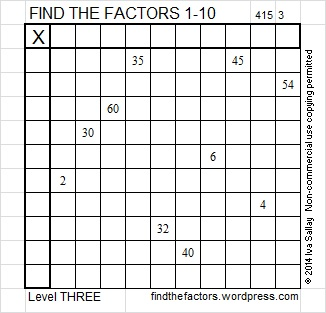# 92 and Level 3

92 is a composite number. 92 = 1 x 92, 2 x 46, or 4 x 23.  Factors of 92: 1, 2, 4, 23, 46, 92. Prime factorization: 92 = 2 x 2 x 23 which can also be written 92 = 2² x 23.92 is never a clue in the FIND THE FACTORS puzzles.This week’s puzzles and last week’s solutions: 10 Factors 2014-04-14

A Logical Approach to find the factors: Find the column or row with two clues and find their common factor. Write the corresponding factors in the factor column and factor row.  Because this is a level three puzzle, you have now written a factor at the top of the factor column. Continue to work from the top of the factor column to the bottom, finding factors and filling in the table one row at a time as you go: